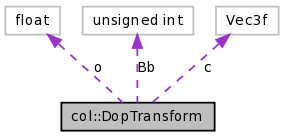# col::DopTransform Struct Reference

Affine transformation for DOP re-alignment. More...

Collaboration diagram for col::DopTransform:[legend]
List of all members.

## Public Member Functions

General functions
DopTransform (const osg::Matrix &m)
Calculate DOP transformation from a matrix.
DopTransform (const osg::Quaternion &q)
This is an overloaded member function, provided for convenience. It differs from the above function only in what argument(s) it accepts.
void operator= (const osg::Matrix &m)
Calculate DOP transformation from a matrix.
void operator= (const osg::Quaternion &q)
This is an overloaded member function, provided for convenience. It differs from the above function only in what argument(s) it accepts.
void print (void) const
Print a DOP transformation.
Dop operator * (const Dop &dop) const
Transform a DOP.

## Public Attributes

Vec3f c [Dop::NumOri]
The transformation matrix.
float o [Dop::NumOri]
The translation of the affine transformation.
unsigned int Bb [Dop::NumOri]
The correspondence between old and new DOP coefficients.

## Detailed Description

Affine transformation for DOP re-alignment.

## Constructor & Destructor Documentation

 col::DopTransform::DopTransform ( const osg::Matrix & m )

Calculate DOP transformation from a matrix.

Parameters:
 m the transformation matrix (rot + transl)
The result can be used for operator* .

Sideeffects:
Class variables m_Ori, m_Vtx2Ori, m_Pnt will be used.
Implementation:
= opyCorrespFromMat in Y.

## Member Function Documentation

 void col::DopTransform::operator= ( const osg::Matrix & m )

Calculate DOP transformation from a matrix.

DopTransform::DopTransform( const osg::Matrix &m )

 Dop col::DopTransform::operator * ( const Dop & dop ) const

Transform a DOP.

Parameters:
 dop a Dop
If the transformation has been computed with DopTransform(osg::Matrix&), then the result will be an axis-aligned DOP enclosing the original one.

Implementation:
= opyCalcPlaneOffsets in Y. It seems that the double indexing costs about half the time!

The documentation for this struct was generated from the following files:

Generated on Tue Oct 16 18:12:50 2007 for CollDet by1.5.2